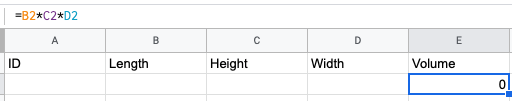# How to insert a new row with data and formulas in Google Sheets

Normally you would add more data by using "Insert a New Row", and is great but, you cannot use formulas at that row, or apply the same formula to the whole column.

However there is a way to do this: ARRAYFORMULA. We've been inspired by an article from Labnol.org that describes a method that will be very handy in Landbot.

In this example we will obtain the volume of a room (m3) based length, height and width (in cms):

1. We set up the Columns of the Spreadsheet

1. The goal is that volume always displays the result of the operation. The standard formula would be:1. As we want to be always the content no matter in which row is the formula should be change to:

1. Here is where we want that the ARRAYFORMULA method is applied to all the column:

1. But we want to apply directly from the first row, so we set up the condition and move the formula up, and set up a condition where we set up that if is the first row, the value of the cell will be "Volume":

1. Now is the important bit to be used in Landbot. Here we add a condition, that specifies that if there is missing a value of Length, do not trigger the formula, so it won't add results when there is no Length value

`=ARRAYFORMULA(IF(ROW(E:E)=1,"Volume",IF(ISBLANK(B:B),"", B:B*C:C*D:D)))`

1. In Landbot now we can Set up the question for the inputs and Google Spreadsheet block to Insert a New Row, where we will add only the Length, Height and Width: